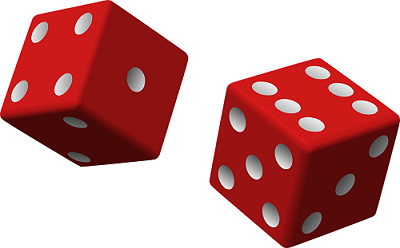# Empirical And Theoretical Probability

Before talking about empirical and theoretical probability, we first recall what probability is. We come across a number of situations every day where we need to predict the outcome of an event before the event has taken place. We often use the statements like ‘it might rain today’ or ‘chances are that I will reach on time’, etc. Thus, there are many events whose outcome cannot be foreseen; they are decided by chance. Let us say, you toss a coin. The chance, or probability, of getting a head is same as that of getting a tail, that is, 50%. In this article, we will discuss more such scenarios and introduce you to the concept of probability as well as empirical and theoretical probability.#### What is Probability?

Probability is the measurement of chances – the likelihood that an event will occur. If the probability of an event is high, it is more likely that the event will happen. It is measured between 0 and 1, inclusive. So if an event is unlikely to occur, its probability is 0 whereas 1 indicates the certainty for the occurrence.

## Empirical Probability V/s Theoretical Probability

Empirical or Experimental probability

When you toss a coin, there are equal chances of a head or a tail to come up. So we say that probability of head or tail is ½ or 0.5 each. Now repeat the experiment with 100 coins. You will observe that frequency of the occurrence of head and tail is, if not exactly, still close to 0.5. Try with 1000 coins. The result may turn out to be head 480/1000 and tail 520/1000. This accounts for being close to 0.5 as well.

This is basically collecting data or running practical experiments to estimate the occurrence of an event. This is called empirical or experimental probability. Thus, the empirical probability is based entirely on experience and observation.

Theoretical Probability

The tossing of coin experiment has been conducted several times in different parts of the world with different number of coins to observe the outcomes. As the number of experiments increased, it turned out that no matter how many coins you toss, the result is always close to ½. So theoretically the probability of getting a head or a tail can be assumed to be 0.5.

Thus, the theoretical probability can be defined as the probability of the occurrence of an event that comes from a sample space of known equally favourable outcomes.

The difference between the empirical and theoretical probability is evident from the above example.